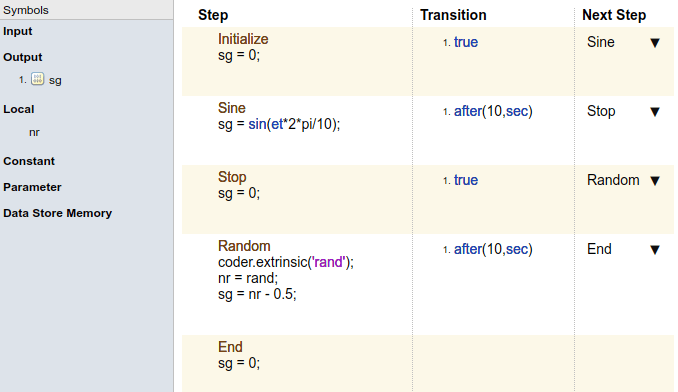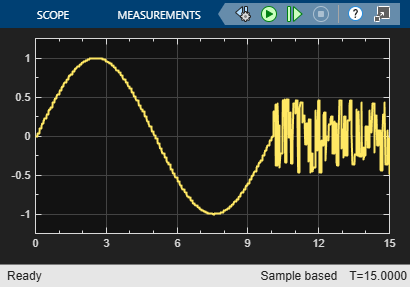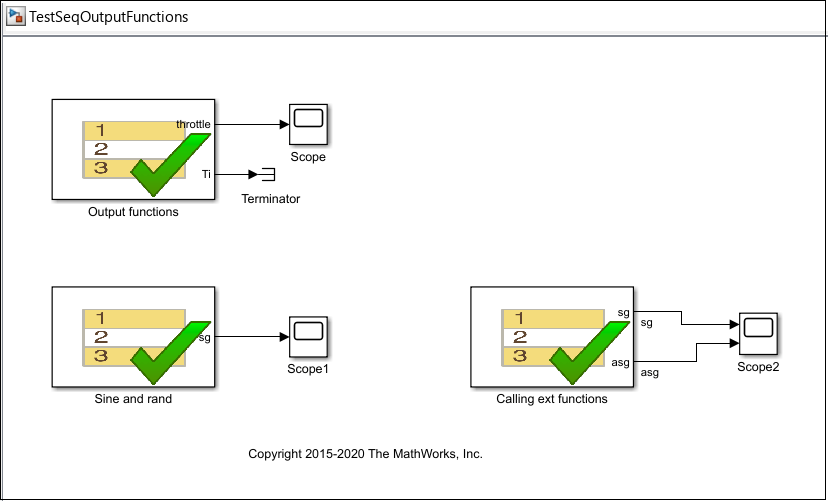Documentation

Signal Generation Functions

In the Test Sequence block, you can generate signals for testing.

1. Define an output data symbol in the Data Symbols pane.

2. Use the output name with a signal generation function in the test step action.

You can call external functions from the Test Sequence block. Define a function in a script on the MATLAB® path, and call the function in the test sequence.

Sinusoidal and Random Number Functions in Test Sequences

This example shows how to produce a sine and a random number test signal in a Test Sequence block.

The step Sine outputs a sine wave with a period of 10 seconds, specified by the argument et*2*pi/10. The step Random outputs a random number in the interval -0.5 to 0.5.The test sequence produces signal sg.Using an External Function from a Test Sequence Block

This example shows how to call an externally-defined function from the Test Sequence block. Define a function in a script on the MATLAB® path, and call the function from the test sequence.

In this example, the step ReducedSine reduces the signal sg using the function Attenuate.The test sequence produces signal sg and attenuated signal asg.Signal Generation Functions

Some signal generation functions use the temporal operator et, which is the elapsed time of the test step in seconds. Scaling, rounding, and other approximations of argument values can affect function outputs. Common signal generation functions include:

FunctionSyntaxDescriptionExample
squaresquare(x)

Represents a square wave output with a period of 1 and range –1 to 1.

Within the interval 0 <= x < 1, square(x) returns the value 1 for 0 <= x < 0.5and –1 for 0.5 <= x < 1.

Output a square wave with a period of 10 sec:

square(et/10)
sawtoothsawtooth(x)

Represents a sawtooth wave output with a period of 1 and range –1 to 1.

Within the interval 0 <= x < 1, sawtooth(x) increases.

Output a sawtooth wave with a period of 10 sec:

sawtooth(et/10)
triangletriangle(x)

Represents a triangle wave output with a period of 1 and range –1 to 1.

Within the interval 0 <= x < 0.5, triangle(x) increases.

Output a triangle wave with a period of 10 sec:

triangle(et/10)
rampramp(x)

Represents a ramp signal of slope 1, returning the value of the ramp at time x.

ramp(et) effectively returns the elapsed time of the test step.

Ramp one unit for every 5 seconds of test step elapsed time:

ramp(et/5)
heavisideheaviside(x)

Represents a heaviside step signal, returning 0 for x < 0 and 1 for x >= 0.

Output a heaviside signal after 5 seconds:

heaviside(et-5)
latchlatch(x)

Saves the value of x at the first time latch(x) evaluates in a test step, and subsequently returns the saved value of x. Resets the saved value of x when the step exits. Reevaluates latch(x) when the step is next active.

Latch b to the value of torque:

b = latch(torque)
sinsin(x)

Returns the sine of x, where x is in radians.

A sine wave with a period of 10 sec:

sin(et*2*pi/10)
coscos(x)

Returns the cosine of x, where x is in radians.

A cosine wave with a period of 10 sec:

cos(et*2*pi/10)
randrand

Uniformly distributed pseudorandom values

Generate new random values for each simulation by declaring rand extrinsic with coder.extrinsic. Assign the random number to a local variable. For example:

coder.extrinsic('rand')
nr = rand
sg = a + (b-a)*nr
randnrandn

Normally distributed pseudorandom values

Generate new random values for each simulation by declaring randn extrinsic with coder.extrinsic. Assign the random number to a local variable. For example:

coder.extrinsic('randn')
nr = randn
sg = nr*2
expexp(x)

Returns the natural exponential function, ${e}^{x}$.

An exponential signal progressing at one tenth of the test step elapsed time:

exp(et/10)